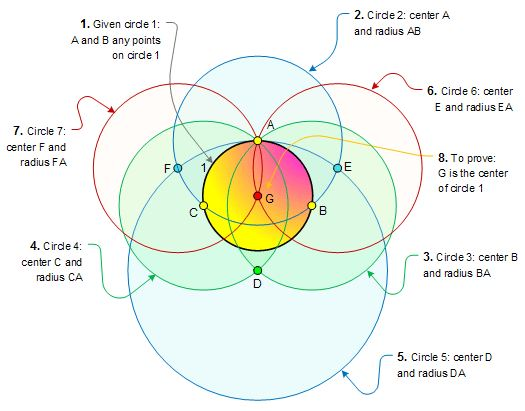## 仅用圆规找圆心

$$\color{red}O$$ 给定仅仅使用圆规找出圆心.

1. 在圆 $$O$$ 上任取一点 $$A$$,以 $$A$$ 为圆心画圆, 交圆 $$O$$ 于 $$B,C$$ 兩点;
2. 分別以 $$B,C$$ 为心,$$AB$$ 为半径画圆交于 $$D$$ 点;
3. 以 $$D$$ 为圆心, $$AD$$ 为半径画圆交圆 $$A$$ 于 $$E,F$$ 两点;
4. 再分别以 $$E,F$$ 为圆心,$$AE$$ 为半径画圆,交于 $$A,G$$, 则 $$G$$ 就是 $$O$$ 点.1. 在已知圆周上任取一点 $$A$$, 以 $$A$$ 为圆心, 适当长为半径作圆 $$A$$, 交已知圆于两点 $$B,C$$;
2. 从 $$B$$ 点出发, 以 $$AB$$ 长为半径, 在圆 $$A$$ 上连续截取 $$3$$ 次得到点 $$D$$;
3. 分别以 $$A,D$$ 为圆心,$$CD$$ 为半径作弧, 两弧交于 $$E$$;
4. 再以 $$E$$ 为圆心, $$EA$$ 为半径作弧交圆 $$A$$ 于 $$F$$;
5. 分别以 $$A,B$$ 为圆心, $$FB$$ 为半径作弧, 两弧的交点就是所求已知圆的圆心.

This site uses Akismet to reduce spam. Learn how your comment data is processed.